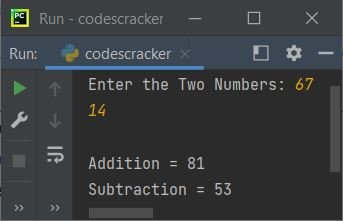# Python from Keyword

The from keyword in Python is used when we need to import a specified section from a module. For example:

```from operator import add

The output is:

`30`

In above program, instead of importing the whole operator module, that contains extensive number of methods, I've imported only the add() one.

And because I've imported only the add() from operator module. Therefore if we access the other methods like sub() of same module, then we will get an error saying that the sub is not defined. For example:

```from operator import add

print(sub(10, 5))```

The snapshot given below shows the output produced by above program:Therefore be sure to import the particular section of a module. That is, only import particular method(s) of a module, if you're sure, not to use any other method(s) of the same module.

But if you replace the following statement, from above program:

`from operator import add`

with the statement given below:

`import operator`

Then the output will be:

`5`

Important - The output will be 5, of course, but you need to use sub() along with operator, in this way:

`print(operator.sub(10, 5))`

## Python from Keyword Example

Let's create another program to demonstrate the from keyword in Python. But before creating the program, that uses from keyword, let's first create a program, without from.

#### Without from Keyword

```import operator

print("Enter the Two Numbers: ", end="")
a = int(input())
b = int(input())

print("Subtraction =", operator.sub(a, b))```

#### With from Keyword

```from operator import add, sub

print("Enter the Two Numbers: ", end="")
a = int(input())
b = int(input())

print("Subtraction =", sub(a, b))```

#### Output of Both the ProgramIn this program, since I've imported the particular two methods of operator module, therefore these two methods are directly called with its name. That is, without using the operator.methodName format.

Python Online Test

« Previous Tutorial Next Tutorial »